# Solution assignment 22 Differentiation of standard functions

### Assignment 22

Differentiate: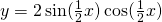### Solution

It is difficult to differentiate this function. It is not in the list of standard functions. Furthermore, there is a product of two trigonometric functions and we do not know how to differentiate this. Finally, the trigonometric functions have an argument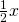.
Yet is appears quite simple to differentiate this function by applying one of the so-called double-angle formulas, i.e. by applying the trigonometric formula: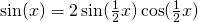So the original function becomes: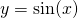which is quite easy to differentiate: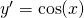0